Next: Applications to Tryptophan Zippers Up: Results Previous: Applications to Isomerisation of   Contents

## A Dijkstra-based Selector

An essential part of the connection algorithm is a mechanism to incorporate the information obtained in all the previous searches into the next one. For large endpoint separations guessing the initial pathway can be difficult, and there is a large probability of finding many irrelevant stationary points at the beginning of the calculation.

The connection algorithm described earlier uses one double-ended search per cycle. However, we have found that this approach can be overwhelmed by the abundance of stationary points and pathways for complicated rearrangements. We therefore introduce the idea of an unconnected pathway and make the connection algorithm more focused by allowing more than one double-ended search per cycle.

Before each cycle a decision must be made as to which minima to try and connect next. Various strategies can be adopted, for example, selection based on the order in which transition states were found , or, selection of minima with the minimal separation in Euclidean distance space . However, when the endpoints are very distant in configuration space, neither of these approaches is particularly efficient. The number of possible connections that might be tried simply grows too quickly if the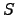,and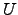sets become large. However, the new algorithm described below seems to be very effective.

The modified connection algorithm we have used in the present work is based on a shortest path method proposed by Dijkstra [154,153]. We can describe the minima that are known at the beginning of each connection cycle as a complete graph ,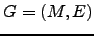, where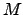is the set of all minima and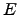is the set of all the edges between them. Edges are considered to exist between every pair of minima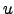and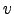, even if they are in different,orsets, and the weight of the edge is chosen to be a function of the minimum Euclidean distance between them :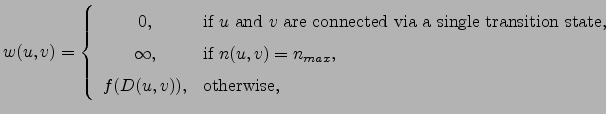(4.44)

where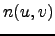is the number of times a pair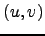was selected for a connection attempt,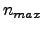is the maximal number of times we may try to connect any pair of minima, and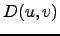is the minimum Euclidean distance betweenand.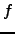should be a monotonically increasing function, such as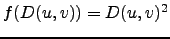. We denote the number of minima in the set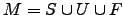, as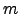, and the number of edges in the setas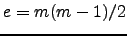.

Using the Dijkstra algorithm [154,153] and the weighted graph representation described above, it is possible to determine the shortest paths between any minima in the database. The source is selected to be one of the endpoints. Upon termination of the Dijkstra algorithm, a shortest path from one endpoint to the other is extracted. If the weight of this pathway is non-zero, it contains one or more `gaps'. Connection attempts are then made for every pairof adjacent minima in the pathway with non-zero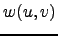using the DNEB approach .

The computational complexity of the Dijkstra algorithm is at worst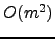, and the memory requirements scale in a similar fashion. The most appropriate data structure is a weighted adjacency matrix. For the calculations presented here, the single source shortest paths problem was solved at the beginning of each cycle, which took less than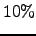of the total execution time for the largest database encountered. We emphasise here that once an initial path has been found, the perturbations considered in typical discrete path sampling (DPS) calculations will generally involve attempts to connect minima that are separated by far fewer elementary rearrangements than the endpoints. It is also noteworthy that the initial path is unlikely to contribute significantly to the overall rate constant. Nevertheless, it is essential to construct such a path to begin the DPS procedure.

The nature of the definition of the weight function allows the Dijkstra algorithm to terminate whenever a second endpoint, or any minimum connected to that endpoint via a series of elementary rearrangements, is reached. This observation reduces the computational requirements by an amount that depends on the distribution of the minima in the database among the,andsets. One of the endpoints is always a member of theset, while the other is a member ofset. Either one can be chosen as the source, and we have found it most efficient to select the one from the set with fewest members. However, this choice does not improve the asymptotic bounds of the algorithm.Next: Applications to Tryptophan Zippers Up: Results Previous: Applications to Isomerisation of   Contents
Semen A Trygubenko 2006-04-10